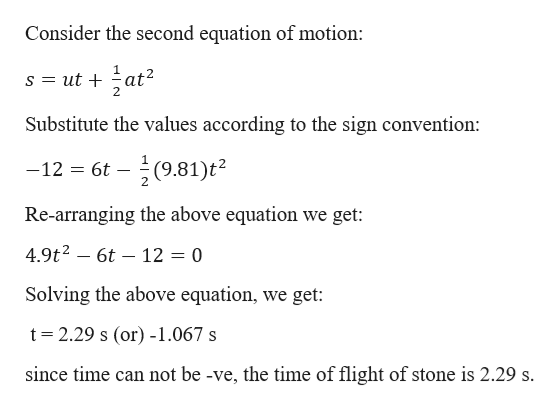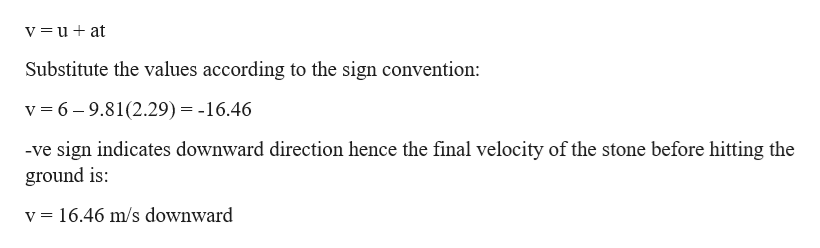You throw a stone vertically upward with an initial speed of 6.0m/s from a third-story office window. If the window is 12m above the ground, find(a) the time the stone is in flight(b)the speed of the stone just before it hits the ground

Question

You throw a stone vertically upward with an initial speed of 6.0m/s from a third-story office window. If the window is 12m above the ground, find

(a) the time the stone is in flight

(b)the speed of the stone just before it hits the ground

Step 1

Given information:

Hight of the window (h) = 12 m.

Initial velocity (u) = 6 m/s

Let us consider the upward direction as +ve and down ward direction as -ve

We know that the object moves under acceleration due to gravity hence (a) = -g

Step 2

Consider the second equation of motion:help_outlineImage TranscriptioncloseConsider the second equation of motion: s ut at2 Substitute the values according to the sign convention: -글(9.81)t2 -12 6t Re-arranging the above equation we get: 4.9t2 6t 12 = 0 Solving the above equation, we get t 2.29 s (or) -1.067 s since time can not be -ve, the time of flight of stone is 2.29 s. fullscreen
Step 3

consider first equati...help_outlineImage TranscriptioncloseV=u + at Substitute the values according to the sign convention: 6-9.81(2.29) = -16.46 v -ve sign indicates downward direction hence the final velocity of the stone before hitting the ground is v = 16.46 m/s downward fullscreen

Want to see the full answer?

See Solution

Want to see this answer and more?

Our solutions are written by experts, many with advanced degrees, and available 24/7

See Solution
Tagged in

Physics